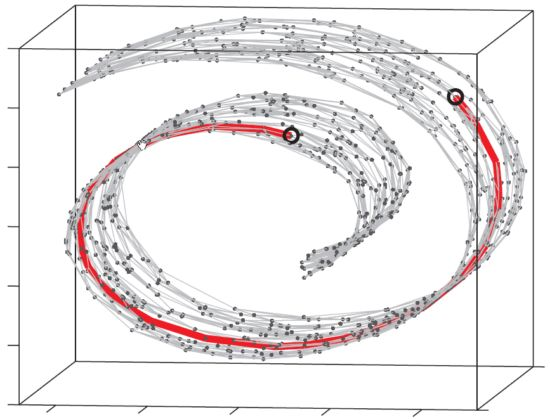# 流形学习的假设

• 所观测到的数据是高维的，高维数据受数据特征的限制会存在冗余信息，实际上用某个低维向量就能得到唯一表示；
• 高维空间的表示有冗余，映射到低维空间的表示没有冗余，低维空间称为“流形空间”。

# 举例：在地球仪上计算北京到上海

• 我们关注地表的距离，而不是三维空间的欧氏距离；

• 从高维空间上看，北京的坐标是$(x_1,y_1,z_1)$，上海的坐标是$(x_2,y_2,z_2)$，采用空间欧式距离两个点之间的距离是$\sqrt{(x_1-x_2)^2+(y_1-y_2)^2+(z_1-z_2)^2}$，但是这种方式度量两个城市之间的距离不准确；

• 把三维的地球展成二维平面，二维平面就是合适的流形空间，此时北京的坐标是$(x_1,y_1)$，上海的坐标是$(x_2,y_2)$，采用空间欧式距离两个点之间的距离是$\sqrt{(x_1-x_2)^2+(y_1-y_2)^2}$，此时使用欧式距离度量才有意义。

# 结论

1、只有流形空间上使用欧式距离才有意义；

2、$m$维流形所展开的$n$维空间$(m<n)$不适合使用欧氏距离。

# 图解二维流形——瑞士卷# 流形学习研究内容

• 在得到模型有效的高维特征表示后，如何实现高维到低维空间的非线性降维，这种降维既能保证能合理使用欧式距离，也能考虑到数据的拓扑结构；
• 如何保证映射的低维空间是流形空间。